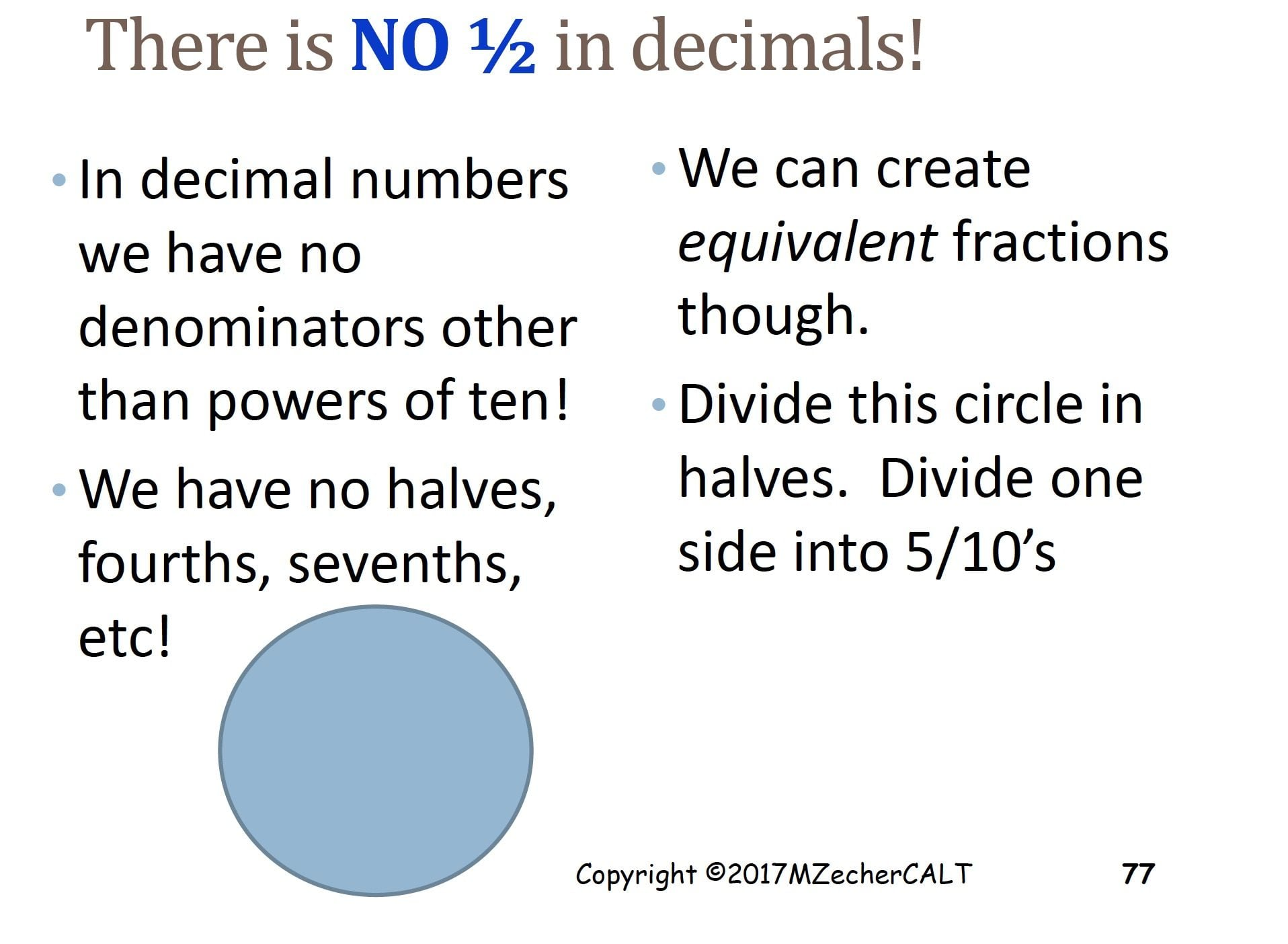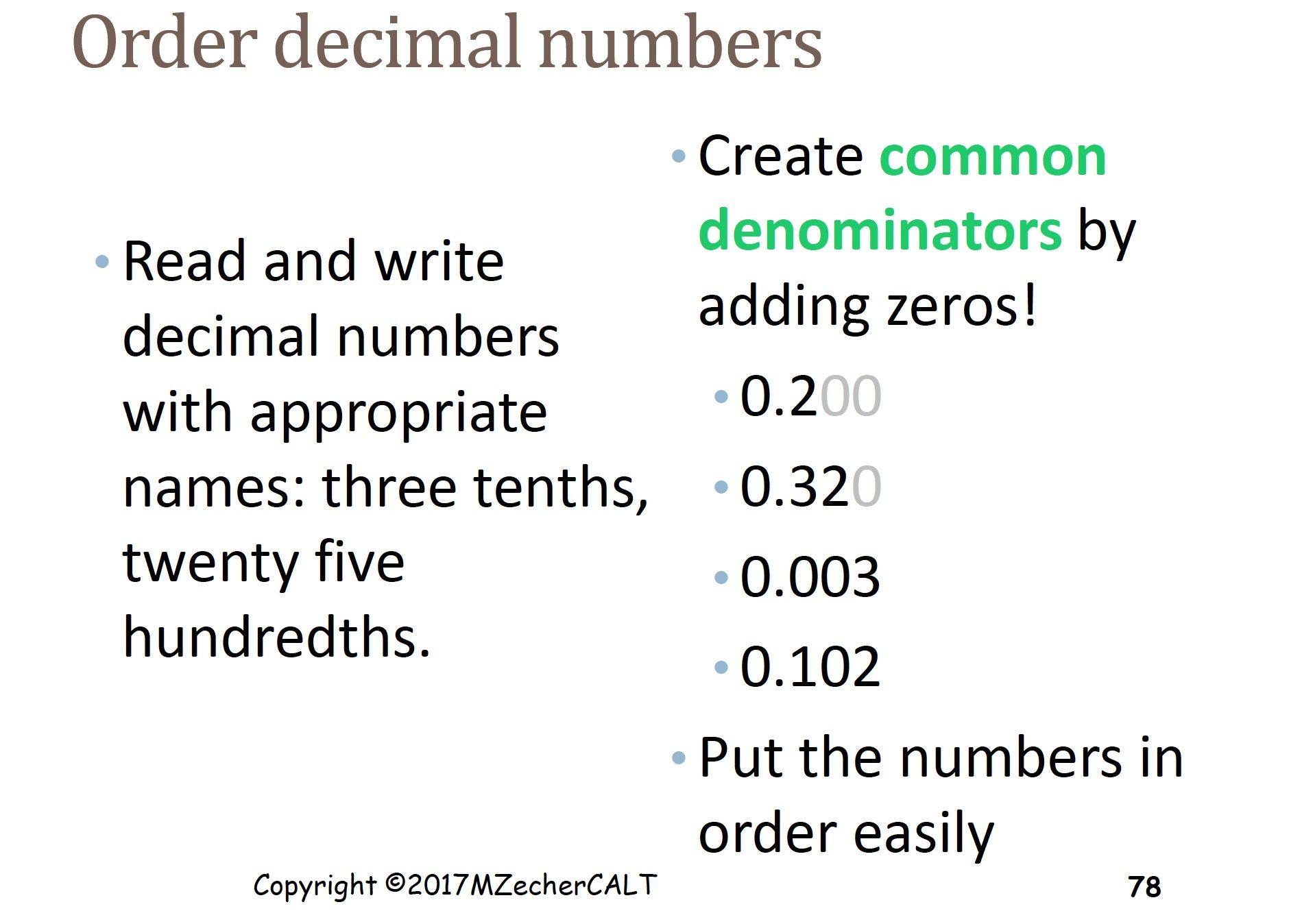### WHY DECIMALS ARE HARD

There are many confusing aspects to decimals that are helpful to recognize when helping students. Contrary to working with whole numbers, longer sequences of numbers are not larger than shorter ones. For instance, with whole numbers, 245 is greater than 2, but .0245 is smaller than 2.

For math processes involving decimals, multiplying by a decimal number between 0 and 1 is also opposite to what one might be used to. After learning that multiplication is equal groups or repeated addition, multiplying 0.3 x 0.4 = 0.12, a number that is smaller than 0.3 or 0.4. Similarly, dividing by a decimal can result in a number that is bigger than what we started out with, which can seem even more confusing.

Even decimal addition and subtraction can seem confusing because numbers need to be lined up by their decimal point which may seem different than lining numbers up by the right hand side.

From Marilyn Zecher:

Clarifying the point that decimals are unique fractions based on multiples of 10 can reduce the likelihood of mistakes in the future.What is helpful about Marilyn’s approach is that time is given for students to process what is meant by the new way of representing numbers as decimals instead of fractions or whole numbers.

Also addressed are ways common denominators can be represented in the decimal system (especially important before progressing to calculation steps that emphasize “lining up the decimals”.

Working with base 10 blocks and area grids can be very important for students in order to develop a solid foundation of the meaning of decimals before progressing onto more complex tasks like comparing decimals, addition, subtracting, multiplication, or division.

In the Khan Academy video below, see how comparing decimals is easier with use of area grids.